Download Presentation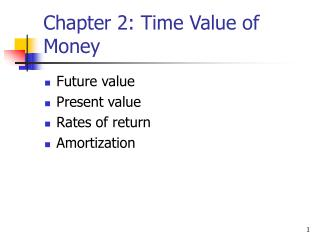Chapter 2: Time Value of Money

# Chapter 2: Time Value of Money - PowerPoint PPT Presentation

Chapter 2: Time Value of Money. Future value Present value Rates of return Amortization. Time lines show timing of cash flows. 0. 1. 2. 3. i%. CF 0. CF 1. CF 2. CF 3. Tick marks at ends of periods, so Time 0 is today; Time 1 is the end of Period 1; or the beginning of Period 2.I am the owner, or an agent authorized to act on behalf of the owner, of the copyrighted work described.
Download Presentation## Chapter 2: Time Value of Money

An Image/Link below is provided (as is) to download presentation

Download Policy: Content on the Website is provided to you AS IS for your information and personal use and may not be sold / licensed / shared on other websites without getting consent from its author.While downloading, if for some reason you are not able to download a presentation, the publisher may have deleted the file from their server.

- - - - - - - - - - - - - - - - - - - - - - - - - - E N D - - - - - - - - - - - - - - - - - - - - - - - - - -
Presentation Transcript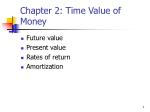Chapter 2: Time Value of Money
• Future value
• Present value
• Rates of return
• Amortization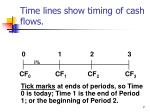Time lines show timing of cash flows.

0

1

2

3

i%

CF0

CF1

CF2

CF3

Tick marks at ends of periods, so Time 0 is today; Time 1 is the end of Period 1; or the beginning of Period 2.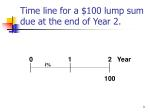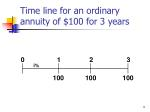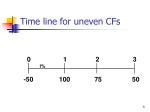Time line for uneven CFs

0

1

2

3

i%

-50

100

75

50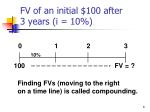FV of an initial \$100 after3 years (i = 10%)

0

1

2

3

10%

100

FV = ?

Finding FVs (moving to the right

on a time line) is called compounding.After 2 years

FV1 = PV + INT1 = PV + PV (i)

= PV(1 + i)

= \$100(1.10)

= \$110.00.

FV2 = FV1(1+i) = PV(1 + i)(1+i)

= PV(1+i)2

= \$100(1.10)2

= \$121.00.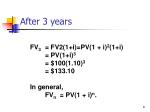After 3 years

FV3 = FV2(1+i)=PV(1 + i)2(1+i)

= PV(1+i)3

= \$100(1.10)3

= \$133.10

In general,

FVn = PV(1 + i)n.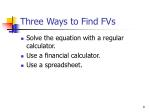Three Ways to Find FVs
• Solve the equation with a regular calculator.
• Use a financial calculator.
• Use a spreadsheet.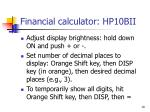Financial calculator: HP10BII
• Adjust display brightness: hold down ON and push + or -.
• Set number of decimal places to display: Orange Shift key, then DISP key (in orange), then desired decimal places (e.g., 3).
• To temporarily show all digits, hit Orange Shift key, then DISP, then =HP10BII (Continued)
• To permanently show all digits, hit ORANGE shift, then DISP, then . (period key)
• Set decimal mode: Hit ORANGE shift, then ./, key. Note: many non-US countries reverse the US use of decimals and commas when writing a number.HP10BII: Set Time Value Parameters
• To set END (for cash flows occurring at the end of the year), hit ORANGE shift key, then BEG/END.
• To set 1 payment per period, hit 1, then ORANGE shift key, then P/YRFinancial Calculator Solution

Financial calculators solve this equation:

FVn + PV (1+i)n = 0.

There are 4 variables. If 3 are known, the calculator will solve for the 4th.Here’s the setup to find FV

INPUTS

3 10 -100 0

N I/YR PV PMT FV

133.10

OUTPUT

Clearing automatically sets everything to 0, but for safety enter PMT = 0.

Set: P/YR = 1, END.• Use the FV function: see spreadsheet in “FM11 Ch 02 Mini Case.xls”
• = FV(Rate, Nper, Pmt, PV)
• = FV(0.10, 3, 0, -100) = 133.10What’s the PV of \$100 due in 3 years if i = 10%?

Finding PVs is discounting, and it’s the reverse of compounding.

0

1

2

3

10%

100

PV = ?n

FVn

(

)

1

PV =

= FVn

(1 + i)n

1 + i

3

1

PV

=

\$100

1.10

=

\$100

0.7513

=

\$75.13.

Solve FVn = PV(1 + i )n for PVFinancial Calculator Solution

INPUTS

3 10 0 100

N I/YR PV PMT FV

-75.13

OUTPUT

Either PV or FV must be negative. Here

PV = -75.13. Put in \$75.13 today, take

out \$100 after 3 years.• Use the PV function: see spreadsheet in “FM11 Ch 02 Mini Case.xls”
• = PV(Rate, Nper, Pmt, FV
• = PV(0.10, 3, 0, 100) = -75.13Finding the Time to Double

0

1

2

?

20%

2

-1

FV = PV(1 + i)n

\$2 = \$1(1 + 0.20)n

(1.2)n = \$2/\$1 = 2

nLN(1.2) = LN(2)

n = LN(2)/LN(1.2)

n = 0.693/0.182 = 3.8.Financial Calculator Solution

INPUTS

20 -1 0 2

N I/YR PV PMT FV

3.8

OUTPUT• Use the NPER function: see spreadsheet in “FM11 Ch 02 Mini Case.xls”
• = NPER(Rate, Pmt, PV, FV)
• = NPER(0.10, 0, -1, 2) = 3.8Finding the Interest Rate

0

1

2

3

?%

2

-1

FV = PV(1 + i)n

\$2 = \$1(1 + i)3

(2)(1/3) = (1 + i)

1.2599 = (1 + i)

i = 0.2599 = 25.99%.Financial Calculator

INPUTS

3 -1 0 2

N I/YR PV PMT FV

25.99

OUTPUT• Use the RATE function:
• = RATE(Nper, Pmt, PV, FV)
• = RATE(3, 0, -1, 2) = 0.2599Ordinary Annuity vs. Annuity Due

Ordinary Annuity

0

1

2

3

i%

PMT

PMT

PMT

Annuity Due

0

1

2

3

i%

PMT

PMT

PMT(1+i)n-1

= PMT

i

(1+0.10)3-1

= 331

= 100

0.10

FV Annuity Formula
• The future value of an annuity with n periods and an interest rate of i can be found with the following formula:(1+i)n-1

FVn + PV(1+i)n + PMT

= 0.

i

Financial Calculator Formula for Annuities
• Financial calculators solve this equation:

There are 5 variables. If 4 are known, the calculator will solve for the 5th.Financial Calculator Solution

INPUTS

3 10 0 -100

331.00

N

I/YR

PV

PMT

FV

OUTPUT

Have payments but no lump sum PV, so enter 0 for present value.• Use the FV function: see spreadsheet.
• = FV(Rate, Nper, Pmt, Pv)
• = FV(0.10, 3, -100, 0) = 331.00What’s the PV of this ordinary annuity?

0

1

2

3

10%

100

100

100

90.91

82.64

75.13

248.69 = PV1

1 -

(1+i)n

= PMT

i

1

1 -

(1+0.10)3

= 100

= 248.69

0.10

PV Annuity Formula
• The present value of an annuity with n periods and an interest rate of i can be found with the following formula:Financial Calculator Solution

INPUTS

3 10 100 0

N

I/YR

PV

PMT

FV

OUTPUT

-248.69

Have payments but no lump sum FV, so enter 0 for future value.• Use the PV function: see spreadsheet.
• = PV(Rate, Nper, Pmt, Fv)
• = PV(0.10, 3, 100, 0) = -248.69PV and FV of Annuity Due vs. Ordinary Annuity
• PV of annuity due:

= (PV of ordinary annuity) (1+i)

= (248.69) (1+ 0.10) = 273.56

• FV of annuity due:

= (FV of ordinary annuity) (1+i)

= (331.00) (1+ 0.10) = 364.1PV of Annuity Due: Switch from “End” to “Begin

INPUTS

3 10 100 0

-273.55

N

I/YR

PV

PMT

FV

OUTPUT

Then enter PV = 0 and press FV to find

FV = \$364.10.Excel Function for Annuities Due
• Change the formula to:

=PV(10%,3,-100,0,1)

• The fourth term, 0, tells the function there are no other cash flows. The fifth term tells the function that it is an annuity due. A similar function gives the future value of an annuity due:

=FV(10%,3,-100,0,1)What is the PV of this uneven cash flow stream?

4

0

1

2

3

10%

100

300

300

-50

90.91

247.93

225.39

-34.15

530.08 = PVFinancial calculator: HP10BII
• Clear all: Orange Shift key, then C All key (in orange).
• Enter number, then hit the CFj key.
• Repeat for all cash flows, in order.
• To find NPV: Enter interest rate (I/YR). Then Orange Shift key, then NPV key (in orange).Financial calculator: HP10BII (more)
• To see current cash flow in list, hit RCL CFj CFj
• To see previous CF, hit RCL CFj –
• To see subsequent CF, hit RCL CFj +
• To see CF 0-9, hit RCL CFj 1 (to see CF 1). To see CF 10-14, hit RCL CFj . (period) 1 (to see CF 11).Input in “CFLO” register:
• CF0 = 0
• CF1 = 100
• CF2 = 300
• CF3 = 300
• CF4 = -50
• Enter I = 10%, then press NPV button to get NPV = 530.09. (Here NPV = PV.)Nominal rate (iNom)
• Stated in contracts, and quoted by banks and brokers.
• Not used in calculations or shown on time lines
• Periods per year (m) must be given.
• Examples:
• 8%; Quarterly
• 8%, Daily interest (365 days)Periodic rate (iPer )
• iPer = iNom/m, where m is number of compounding periods per year. m = 4 for quarterly, 12 for monthly, and 360 or 365 for daily compounding.
• Used in calculations, shown on time lines.
• Examples:
• 8% quarterly: iPer = 8%/4 = 2%.
• 8% daily (365): iPer = 8%/365 = 0.021918%.Will the FV of a lump sum be larger or smaller if we compound more often, holding the stated I% constant?
• LARGER!
• If compounding is more frequent than once a year--for example, semiannually, quarterly, or daily--interest is earned on interest more often.mn

i

Nom

FV

=

PV

1 .

+

n

m

2x5

0.12

FV

=

\$100

1

+

5S

2

= \$100(1.06)10 = \$179.08.

FV with Different Compounding Periods (e.g., \$100 at a 12% nominal rate with semiannual compounding for 5 years)FV(Annual)= \$100(1.12)5 = \$176.23.

FV(Semiannual)= \$100(1.06)10=\$179.08.

FV(Quarterly)= \$100(1.03)20 = \$180.61.

FV(Monthly)= \$100(1.01)60 = \$181.67.

FV(Daily) = \$100(1+(0.12/365))(5x365)

= \$182.19.Effective Annual Rate (EAR = EFF%)
• The EAR is the annual rate which causes PV to grow to the same FV as under multi-period compounding. Example: Invest \$1 for one year at 12%, semiannual:

FV = PV(1 + iNom/m)m

FV = \$1 (1.06)2 = 1.1236.

• EFF% = 12.36%, because \$1 invested for one year at 12% semiannual compounding would grow to the same value as \$1 invested for one year at 12.36% annual compounding.Comparing Rates
• An investment with monthly payments is different from one with quarterly payments. Must put on EFF% basis to compare rates of return. Use EFF% only for comparisons.
• Banks say “interest paid daily.” Same as compounded daily.m

EFF% = - 1

(1 + )

iNom

m

(1 + )

2

0.12

2

= - 1.0

= (1.06)2 - 1.0

= 0.1236 = 12.36%.

EFF% for a nominal rate of 12%, compounded semiannuallyFinding EFF with HP10BII
• Type in nominal rate, then Orange Shift key, then NOM% key (in orange).
• Type in number of periods, then Orange Shift key, then P/YR key (in orange).
• To find effective rate, hit Orange Shift key, then EFF% key (in orange).EAR (or EFF%) for a Nominal Rate of of 12%

EARAnnual = 12%.

EARQ = (1 + 0.12/4)4 - 1 = 12.55%.

EARM = (1 + 0.12/12)12 - 1 = 12.68%.

EARD(365) = (1 + 0.12/365)365 - 1 = 12.75%.Can the effective rate ever be equal to the nominal rate?
• Yes, but only if annual compounding is used, i.e., if m = 1.
• If m > 1, EFF% will always be greater than the nominal rate.When is each rate used?

iNom:

Written into contracts, quoted by banks and brokers. Not used in calculations or shown

on time lines.When is each rate used? (Continued)

iPer:

Used in calculations, shown on time lines.

If iNom has annual compounding,

then iPer = iNom/1 = iNom.When is each rate used? (Continued)
• EAR (or EFF%): Used to compare returns on investments with different payments per year.
• Used for calculations if and only if dealing with annuities where payments don’t match interest compounding periods.Amortization
• Construct an amortization schedule for a \$1,000, 10% annual rate loan with 3 equal payments.Step 1: Find the required payments.

0

1

2

3

10%

-1,000

PMT

PMT

PMT

3 10 -1000 0

INPUTS

N

I/YR

PV

FV

PMT

OUTPUT

402.11Find interest charge and principal repayment for Year 1.

Step 1: Find interest

INTt = Beg balt (i)

INT1 = \$1,000(0.10) = \$100.

Step 2: Find repaid princ.

Repmt = PMT - INT

= \$402.11 - \$100

= \$302.11.Step 4: Find ending balance after Year 1.

End bal = Beg bal - Repmt

= \$1,000 - \$302.11 = \$697.89.

Repeat these steps for Years 2 and 3

to complete the amortization table.Amortization tables are widely used--for home mortgages, auto loans, business loans, retirement plans, and more. They are very important!
• Financial calculators (and spreadsheets) are great for setting up amortization tables.Fractional Time Periods
• On January 1 you deposit \$100 in an account that pays a nominal interest rate of 11.33463%, with daily compounding (365 days).
• How much will you have on October 1, or after 9 months (273 days)? (Days given.)Convert interest to daily rate

iPer = 11.33463%/365

= 0.031054% per day.

0

1

2

273

0.031054%

FV=?

-100Calculator Solution

iPer = iNom/m

= 11.33463/365

= 0.031054% per day.

INPUTS

273 -100 0

108.85

I/YR

PV

FV

N

PMT

OUTPUTWhat’s the value at the end of Year 3 of the following CF stream if the quoted interest rate is 10%, compounded semiannually?

4

5

0

1

2

3

6 6-mos.

periods

5%

100

100

100Non-matching rates and periods
• Payments occur annually, but compounding occurs each 6 months.
• So we can’t use normal annuity valuation techniques.1st Method: Compound Each CF

0

1

2

3

4

5

6

5%

100

100.00

100

110.25

121.55

331.80

FVA3 = \$100(1.05)4 + \$100(1.05)2 + \$100

= \$331.80.Use EAR = 10.25% as the annual rate in calculator.

INPUTS

3 10.25 0 -100

N

PV

FV

I/YR

PMT

OUTPUT

331.80What’s the PV of this stream?

0

1

2

3

5%

100

100

100

90.70

82.27

74.62

247.59Comparing Investments
• You are offered a note which pays \$1,000 in 15 months (or 456 days) for \$850. You have \$850 in a bank which pays a 6.76649% nominal rate, with 365 daily compounding, which is a daily rate of 0.018538% and an EAR of 7.0%. You plan to leave the money in the bank if you don’t buy the note. The note is riskless.
• Should you buy it?Daily time line

iPer = 0.018538% per day.

0

365

456 days

-850

1,000Method 1: Greatest Future Wealth

Find FV of \$850 left in bank for

15 months and compare with

note’s FV = \$1,000.

FVBank = \$850(1.00018538)456

= \$924.97 in bank.

Buy the note: \$1,000 > \$924.97.Calculator Solution to FV

iPer = iNom/m

= 6.76649%/365

= 0.018538% per day.

INPUTS

456 -850 0

924.97

N

I/YR

PV

FV

PMT

OUTPUTMethod 2: Greatest Present Wealth

Find PV of note, and compare

with its \$850 cost:

PV = \$1,000/(1.00018538)456

= \$918.95.Financial Calculator Solution

6.76649/365 =

INPUTS

456 .018538 0 1000

-918.95

N

I/YR

PV

FV

PMT

OUTPUT

PV of note is greater than its \$850 cost, so buy the note. Raises your wealth.Method 3: Rate of Return

Find the EFF% on note and compare with 7.0% bank pays, which is your opportunity cost of capital:

FVn = PV(1 + i)n

\$1,000 = \$850(1 + i)456

Now we must solve for i.Calculator Solution

INPUTS

456 -850 0 1000

0.035646%

per day

FV

N

I/YR

PV

PMT

OUTPUT

Convert % to decimal:

Decimal = 0.035646/100 = 0.00035646.

EAR = EFF% = (1.00035646)365 - 1

= 13.89%.Using Interest Conversion

P/YR = 365

NOM% = 0.035646(365) = 13.01

EFF% = 13.89

Since 13.89% > 7.0% opportunity cost,